## Want to keep learning?

This content is taken from the Raspberry Pi Foundation & National Centre for Computing Education's online course, Programming 101: An Introduction to Python for Educators. Join the course to learn more.
2.2

## Raspberry Pi Foundation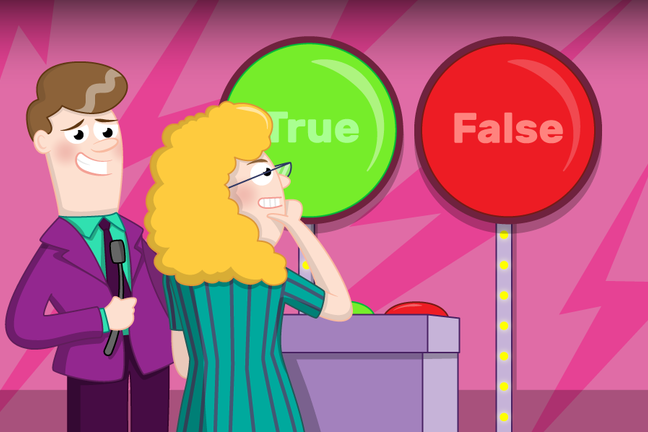# True or False

Computers make choices by determining if the value of a statement is True or False. This is known as evaluation. If the statement is True then the program will run the next instruction, otherwise it won’t.

You can try out some evaluations by using the REPL, also called the console in Trinket. The REPL allows you to run one line of code at a time and see the result immediately. It’s a great tool for testing to see how something works.

Tip: REPL stands for “Read-Eval-Print Loop”. it reads one line of code, evaluates it, prints the result and loops back to the start.

### Mu

If you are using Mu, follow these instructions to use the REPL:

• Open Mu and click on the “REPL” button.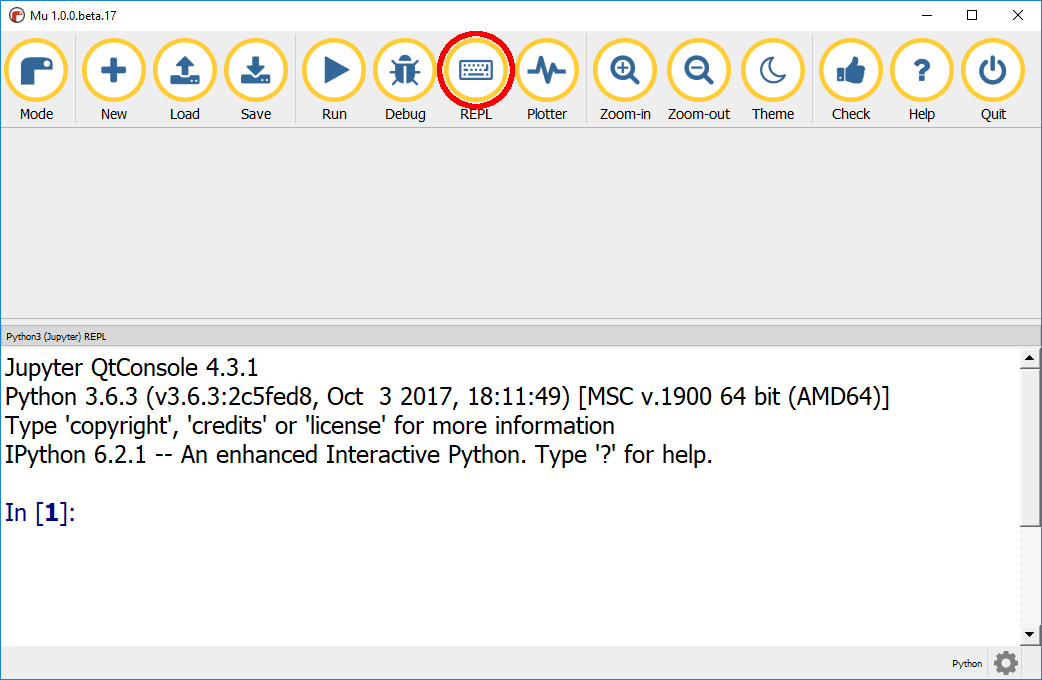### Trinket

If you are using Trinket, follow these instructions to use the REPL (known as console):

• Open trinket.io.

• Create a new Python trinket, by clicking New Trinket, Python.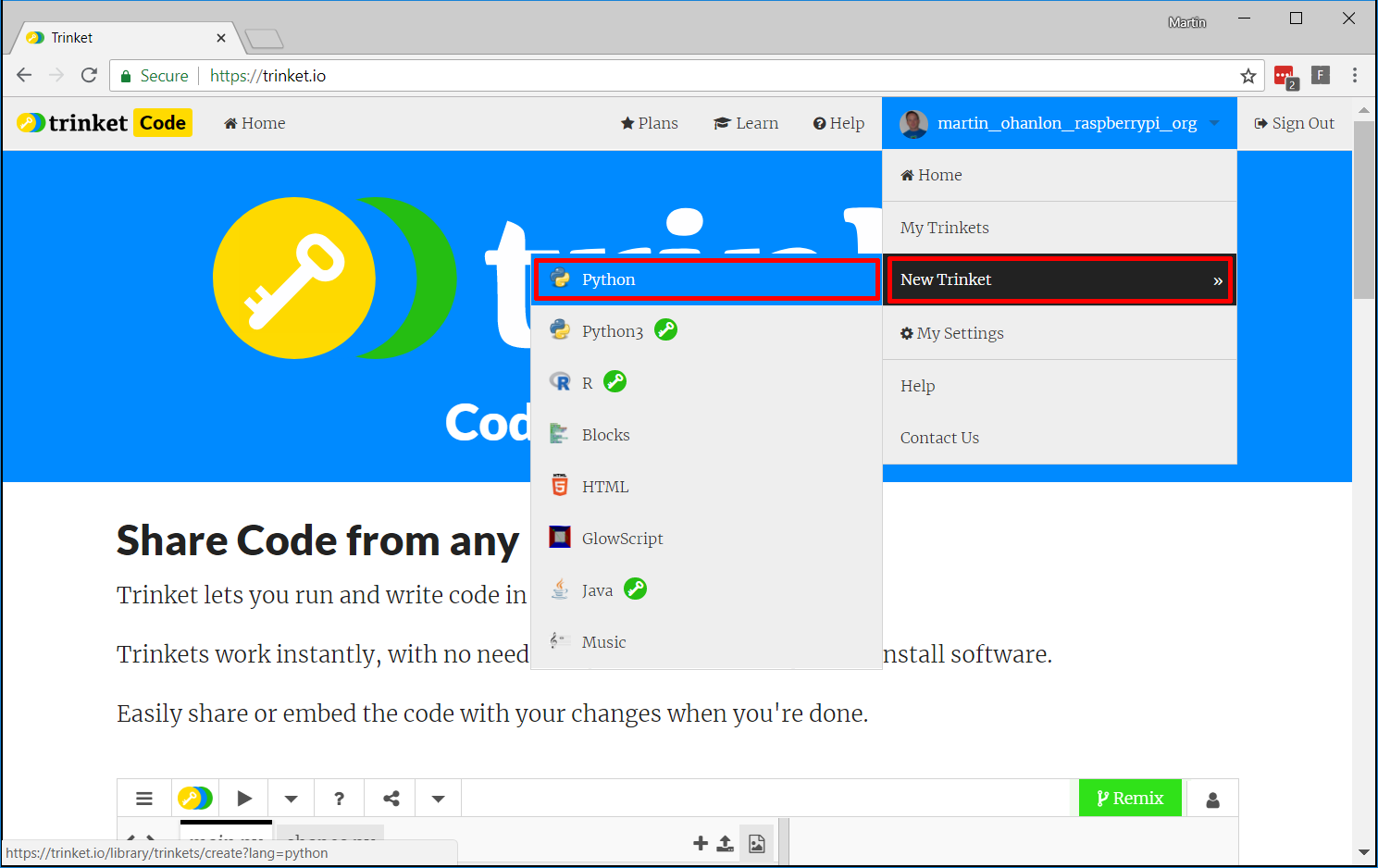• Open the console by clicking on the down arrow next to Play and selecting >_ Console.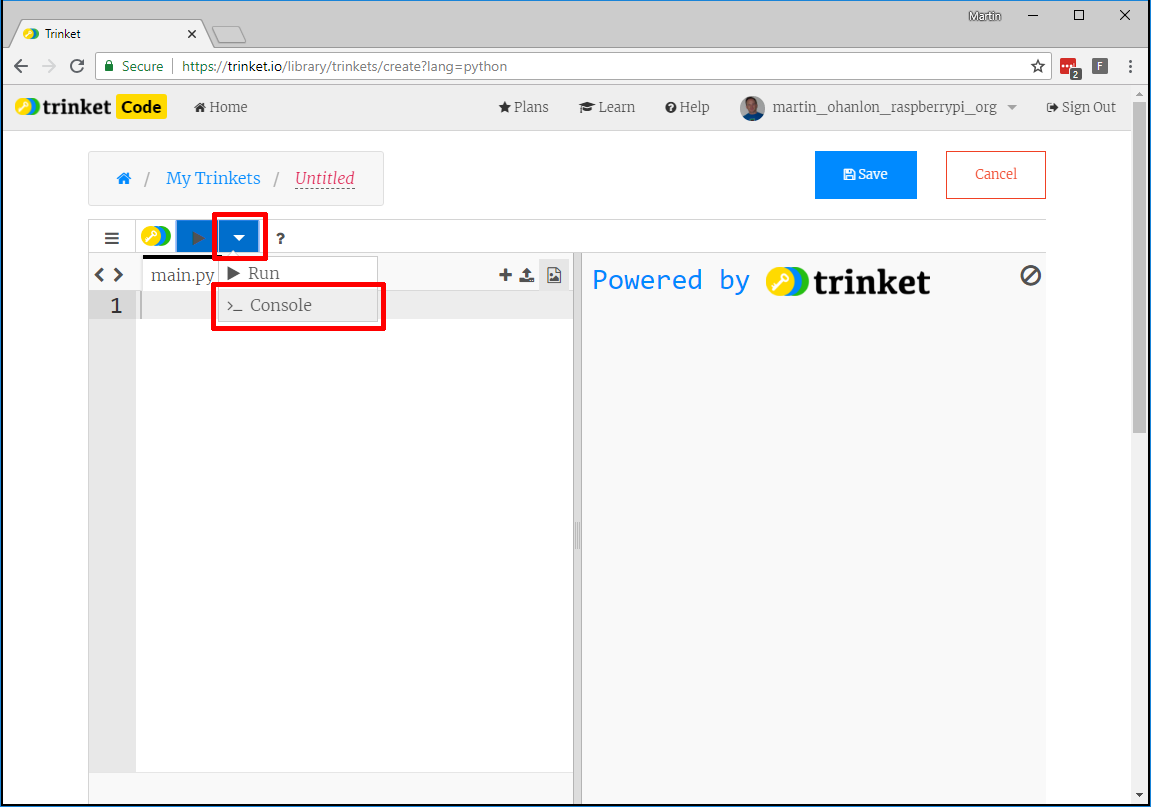### Try some evaluations

• Enter the following into the REPL window and press Enter:
1 == 1


Note: the double == operator means “is this equal to?” as opposed to a single = which follows a variable and means “make this equal to”.

When you press Enter, True will be displayed. This is because it’s true that 1 is equal to 1.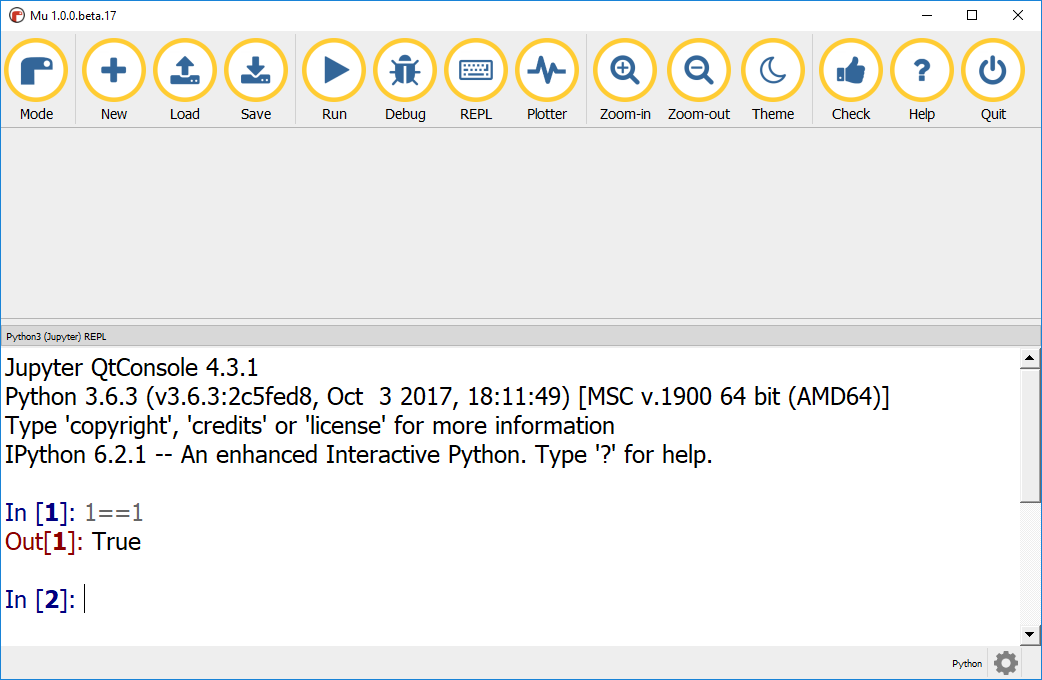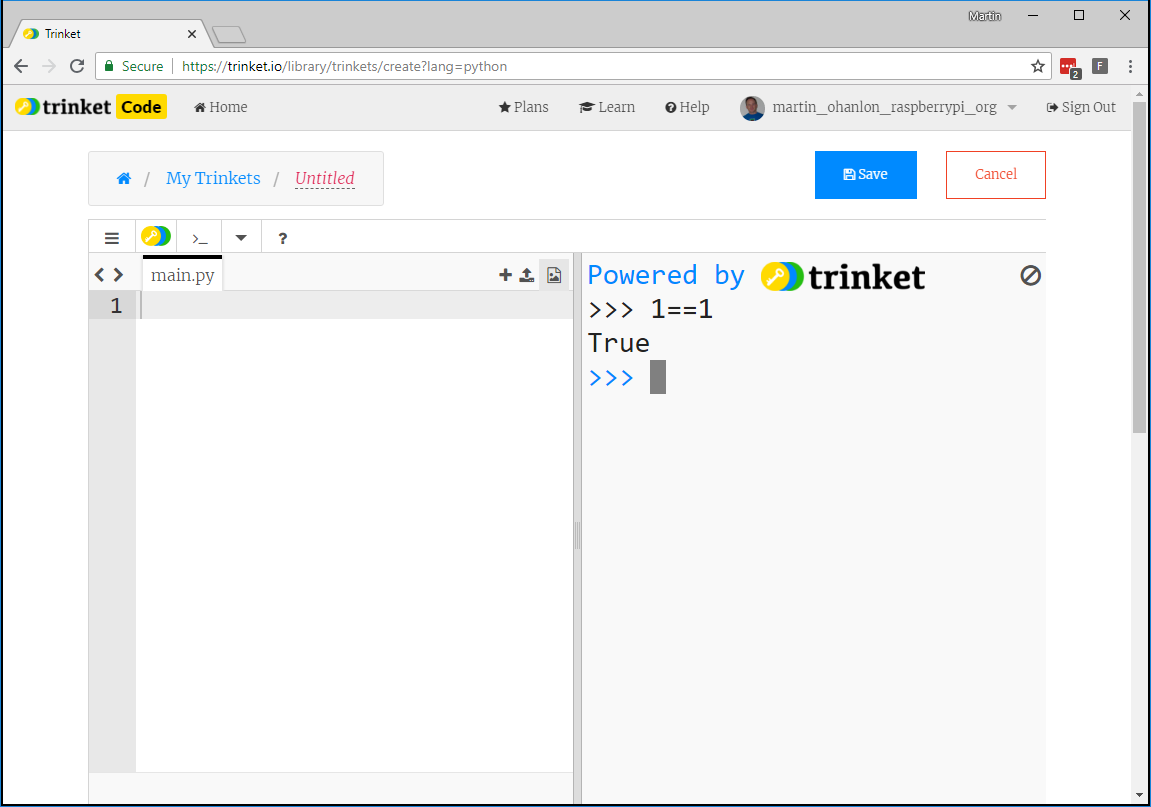• Try entering 1 == 2. False will be displayed, because it’s false that 1 is equal to 2.

• Enter these evaluations and see if you can predict whether the outcome will be True or False.

1 < 2


The operator < means less than. Ask yourself is 1 less than 2? If the answer is yes then the statement is true.

1 > 2


> means greater than, so is 1 greater than 2?

1 != 2


!= is the operator for is not equal to, which means this statement means is 1 not equal to 2. != is the opposite of the == operator.

Whats do you think will be the result of:

1 < 1


This evaluates as false because 1 is not less than 1. However, instead of <, you can use <= test if something is less than or equal to:

1 <= 1


This would give True.

You can evaluate other data types, not just integers (whole numbers) as you have been doing.

• Try some string evaluations:
"hi" == "bye"

"hi" != "bye"


What do you think the result would be for:

"a" < "b"

• Run it in the REPL and observe the result.

Perhaps the result makes sense to you, but what about other character evaluations such as "=" < "!"? Discuss the results in the comments and try to draw some conclusions as to why you get the results you do.

Evaluation is one of the core concepts of computer programming, so it’s important that you understand the principle. Throughout this course there will be lots of opportunities to practise using and creating statements to be evaluated.

You can close the REPL by clicking on the REPL button in Mu or by selecting run from the drop-down menu in Trinket.